# Civil Engineering - Applied Mechanics

### Exercise :: Applied Mechanics - Section 4

46.

A glass ball is shot to hit a wall from a point on a smooth floor. If the ball returns back to the point of projection in twice the time taken in reaching the wall, the coefficient of restitution between the glass ball and the wall is

 A. 0.25 B. 0.33 C. 0.4 D. 0.5 E. 0.55

Explanation:

No answer description available for this question. Let us discuss.

47.

In case of S.H.M. the period of oscillation(T), is given by

 A.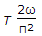B.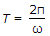C.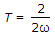D.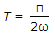Explanation:

No answer description available for this question. Let us discuss.

48.

Kinetic friction may be defined as

 A. friction force acting when the body is just about to move B. friction force acting when the body is in motion C. angle between normal reaction and resultant of normal reaction and limiting friction D. ratio of limiting friction and normal reaction.

Explanation:

No answer description available for this question. Let us discuss.

49.

If α and u are angle of projection and initial velocity of a projectile respectively, the total time of flight, is given by

 A.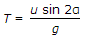B.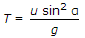C.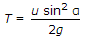D.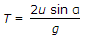Explanation:

No answer description available for this question. Let us discuss.

50.

ω rad/sec is the angular velocity of a crank whose radius is r. If it makes θ° with inner dead centre and obliquity of the connecting rod l is φ, the velocity v of the piston, is given by the equation

 A. ω2(l cos φ + r sin φ tan θ) B. ω2(l sin φ + r cos φ tan θ) C. ω(l sin φ + r cos φ tan θ) D. ω2(l sin φ - r cos θ tan φ).